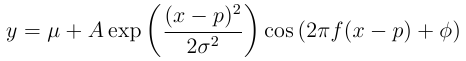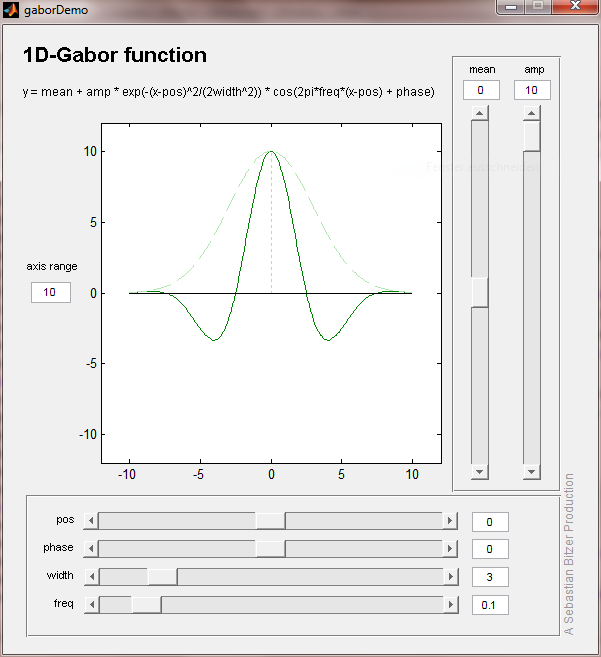# Demos

Working on different projects I have written simple Matlab demos which helped me to understand certain mathematical concepts or models by enabling me to easily manipulate their parameters and observe the resulting changes. Here I provide the Matlab code of the demos and some explanations in the hope that they are helpful to a wider audience. Click on the screenshots to download the corresponding demo!

## 1D Gabor Filter

In neuroscience Gabor filters have been proposed as a model for the receptive fields of neurons in early visual cortex. These filters are defined in two dimensions, but many properties of the Gabor filter can already be understood in one dimension. So here is a Matlab GUI which demonstrates how the 1D Gabor filter can be manipulated. The underlying function is:It consists of two main parts: an exponential which has the same form as a Gaussian function and a cosine which provides a periodic component. The parameters are the mean, mu, the amplitude, A, the position, p, the width of the exponential envelope, sigma, the frequency, f, and the phase, phi, of the periodic component. In the demo the Gabor filter is shown in dark green. Additionally, the exponential envelope and its centre are indicated with light green curves.